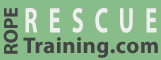# Calculating Mechanical Advantage

This page provides an overview on calculating the mechanical advantage of rope and pulley systems. Upcoming pages explain how to determine the mechanical advantage using the "T-System" and by "Counting the Lines."

Determining the mechanical advantage of a system isn't always obvious. For example, although the following two systems each have one pulley and they look very similar, the first is a 2:1 (you will need to pull with 50 kg of force to raise the 100 kg load) whereas the second is a 1:1 system (you will need to pull 100 kg).

One way to calculate the mechanical advantage is to determine how much rope you would need to pull to raise the load and compare this to how far the load travels. In this first system, you can see that we have to pull 20 feet of rope to raise the load 10 feet—a 2:1 system (i.e., 20 divided by 10 is 2). In the second system, we only need to pull 10 feet to raise the load 10 feet—a 1:1 system.

Working with PowerPoint?
Let vRigger help.